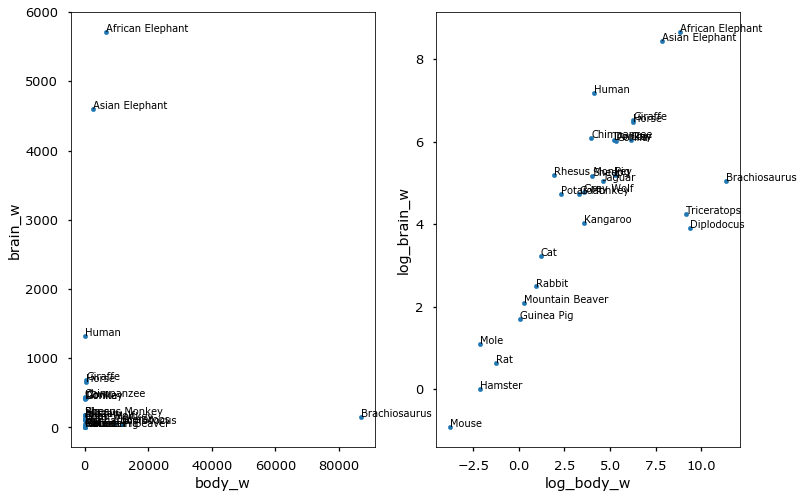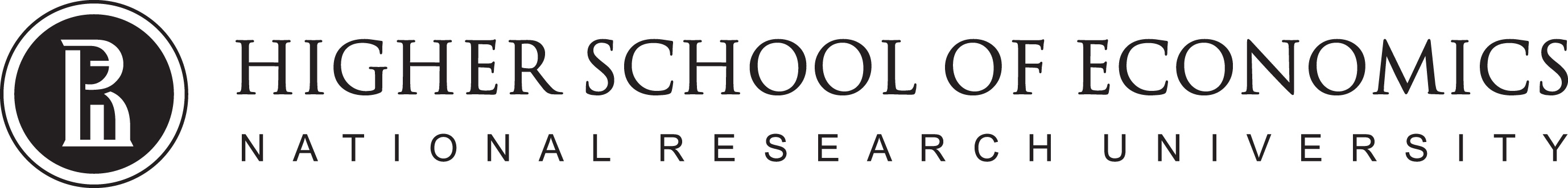# Data Analysis

## Linear regression. Gradient-based optimization1

1. Some materials are taken from machine learning course of Victor Kitov

# Let's recall previous lecture¶

• Decision trees
• Utilize the notion of impurity
• Work both for classification and regression
• Implicit feature selection
• good for features of different nature
• Parallel to axes class separating boundary
• Local greedy optimizaion
• Sensitive to even tiny data pertubations

# Linear regression¶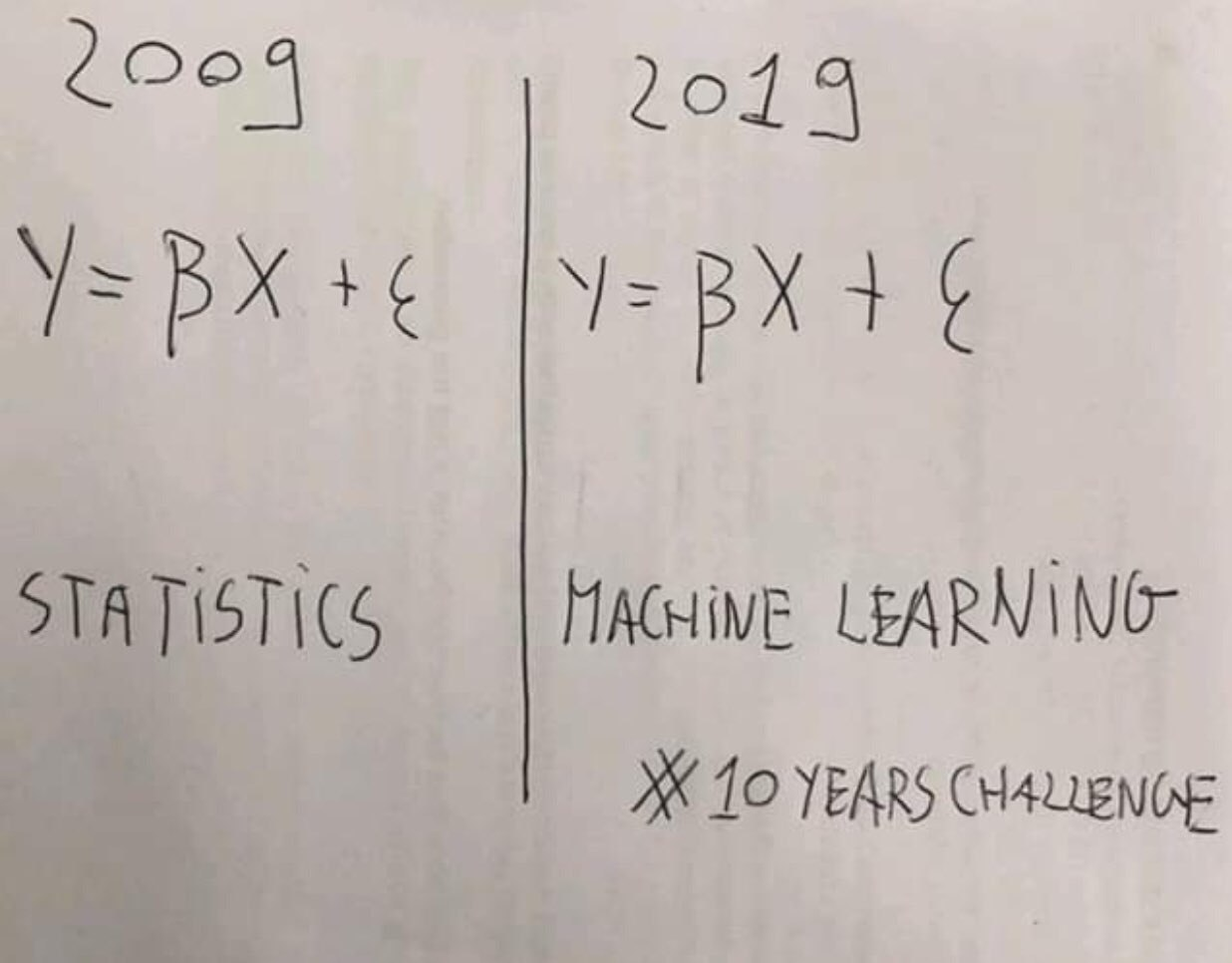## Example: flat prices¶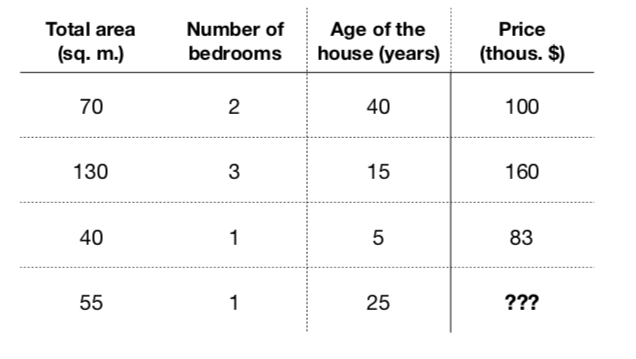• Obviously, those characteristics somehow relate with price ($f: X \rightarrow Y$)
• Formalize a model to predict flat price: $$a(x) = a(total\_area, nmbr\_of\_bedrooms, house\_age) = \hat{y}$$
• Let it be a linear model: $$a(x) = \beta_0 + \beta_1\cdot total\_area + \beta_2 \cdot nmbr\_of\_bedrooms + \beta_3 \cdot house\_age$$
• Learning - find coefficients $\beta_0,\dots, \beta_3$, that minimizer error on training set

## Cars price vs mileage¶

In :
df_auto.plot(x='mileage', y='price', kind='scatter')

Out:
<matplotlib.axes._subplots.AxesSubplot at 0x109dafc88>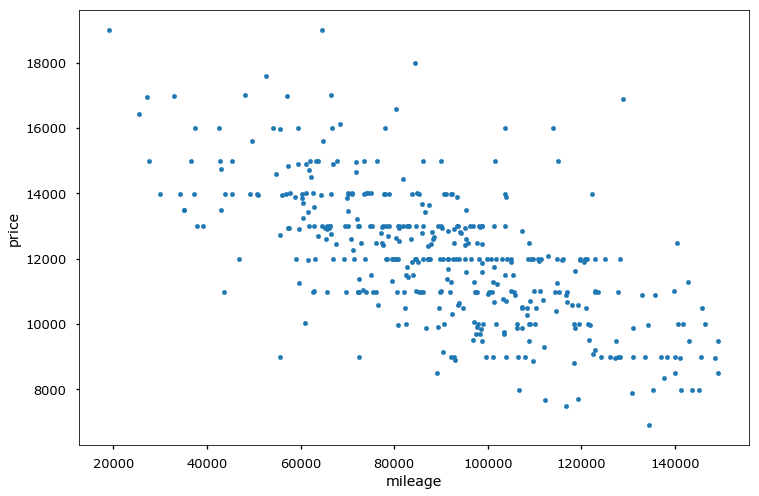## Linear regression¶

• Our goal - determine linear dependence between features $X$ and target vector $y$
• Define $x_n^d$ as $d$-th feature of $n$-th object, $y_{n} \in \mathbb{R}$ - target value for $n$-th object $$f(x_{n}, \beta) = \hat{y}_{n} = \beta_0 + \beta_1x_{n}^1 + \dots$$
• $x_{n}^0 = 1$ $\forall n$ - intercept
• Need to estimate $\beta_i$.

Ordinary Least Squares: $$L(\beta_0,\beta_1,\dots) = \frac{1}{2N}\sum^{N}_{n=1}(\hat{y}_{n} - y_{n})^2 = \frac{1}{2N}\sum^{N}_{n=1}\left(\sum_{d=0}^{D}\beta_{d}x_{n}^{d}-y_{n}\right)^{2} \rightarrow \min\limits_{\beta}$$

## Solution¶

Let's say $f(x, \beta) = \beta_0 + \beta_1x_1$

Calculate partial derivatives wrt $\beta_0$, $\beta_1$ and set them to $0$:

$$\frac{\partial L}{\partial \beta_0} = \frac{1}{N}\sum^{N}_{n=1}(\beta_0 + \beta_1x_{n}^1 - y_{n}) = 0$$$$\frac{\partial L}{\partial \beta_1} = \frac{1}{N}\sum^{N}_{n=1}(\beta_0 + \beta_1x_{n}^1 - y_{n})x^1_{n} = 0$$

## Linear regression (in matrix form)¶

• Define $X\in\mathbb{R}^{NxD},\{X\}_{ij}$ defines the $j$-th feature of $i$-th object, $y\in\mathbb{R}^{n}$ - vector with target values $$f(x, \beta) = \hat{y} = X\beta \quad \Leftrightarrow \quad \left( \begin{array}{c} \hat{y}_1 \\ \hat{y}_2 \\ \vdots \\ \hat{y}_n \end{array} \right) = \left( \begin{array}{ccccc} 1 & x_1^1 & x_1^2 & \cdots & x_1^d\\ 1 & x_2^1 & x_2^2 & \cdots & x_2^d\\ \cdots & \cdots & \cdots & \cdots & \cdots\\ 1 & x_n^1 & x_n^2 & \cdots & x_n^d\end{array} \right) \cdot \left( \begin{array}{c} \beta_0 \\ \beta_1 \\ \vdots\\ \beta_d \end{array} \right)$$
• Need to estimate $\beta_i$.

Ordinary Lears Squares: $$L(\beta) = \frac{1}{2N}(\hat{y} - y)^{\top}(\hat{y} - y) = \frac{1}{2N}(X\beta - y)^{\top}(X\beta - y) \rightarrow \min\limits_{\beta}$$

## Solution (in matrix form)¶

Expand a bit \begin{align*} L(\beta) & = \frac{1}{2N}(X\beta - y)^{\top}(X\beta - y) \\ & = \frac{1}{2N}\left( \beta^\top X^\top X \beta - 2 (X\beta)^\top y + y^\top y \right) \end{align*}

Calculate vector of partial derivatives - gradient

\begin{align*} \nabla L(\beta) = \left(\frac{\partial L(\beta)}{\partial\beta_i} \right)_{i=0\dots d} & = \frac{1}{2N}\left(2X^\top X \beta -2X^\top y\right) = 0 \\ X^{\top}X\beta-X^{T}y & = 0 \\ \\ \beta & = (X^\top X)^{-1} X^\top y \quad\text{(Normal Equation)} \end{align*}

## Comments¶

• This is the global minimum, because the optimized criteria is convex.
• Geometric interpretation:
• find linear combination of feature measurements that best reproduce $y$
• solution - combinaton of features, giving projection of $y$ on linear span of feature measurements.
• Why using Normal Equation could be bad?
• Calculating inverse costs a lot
• Not all matrices have it (singular matrices)

## Linearly dependent features (multicollinearity)¶

• Solution $\widehat{\beta}=(X^{T}X)^{-1}X^{T}y$ exists when $X^{T}X$ is non-singular
• Problem occurs when one of the features is a linear combination of the other (linear dependency)
• interpretation: non-identifiability of $\widehat{\beta}$ for linearly dependent features:
• linear dependence: $\exists\alpha:\,x^{T}\alpha=0\,\forall x$
• suppose $\beta$ solves linear regression $y=x^{T}\beta$
• then $x^{T}\beta\equiv x^{T}\beta+kx^{T}\alpha\equiv x^{T}(\beta+k\alpha)$, so $\beta+k\alpha$ is also a solution!
• Multicollinearity can be exact and not exact, which is also bad

## Examples of linear dependency¶

• $x$ miles $\approx 1.6\cdot x$ kms
• total flat area $\approx$ area of living rooms $+$ area of kitchen
• dummy variable trap!

## Linearly dependent features¶

• Problem may be solved by:
• feature selection
• dimensionality reduction
• imposing additional requirements on the solution (regularization)

## Analysis of linear regression¶

Advantages:

• single optimum, which is global (for non-singular matrix)
• analytical solution
• interpretable solution and algorithm

Drawbacks:

• too simple model assumptions (may not be satisfied)
• $X^{T}X$ should be non-degenerate (and well-conditioned)

# Optimization methods for LR¶

## Intuition¶

$$L(\beta_0, \beta_1) = \frac{1}{2N}\sum_{n=1}^N(\beta_0 + \beta_1x^1_{n} - y_{n})^2$$

• Suppose we have some initial approximation of $(\hat{\beta_0}, \hat{\beta_1})$
• How should we change it in order to improve?
In :
sq_loss_demo()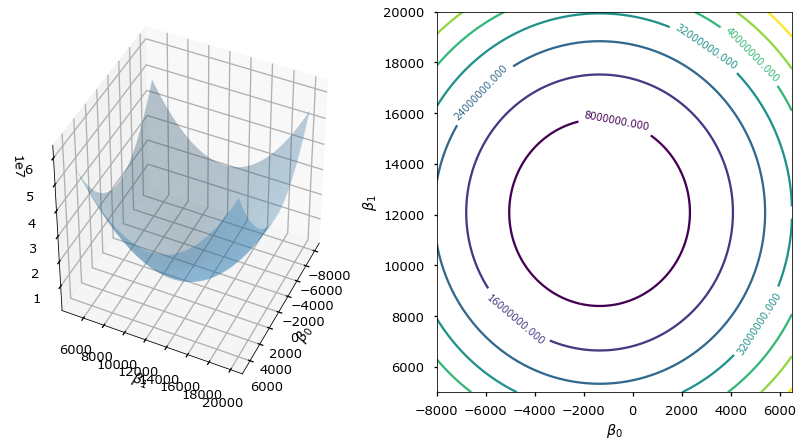## Tiny Refresher¶

Derivative of $f(x)$ in $x_0$: $$f'(x_0) = \lim\limits_{h \rightarrow 0}\frac{f(x_0+h) - f(x_0)}{h}$$

Derivative shows the slope of tangent line in $x_0$

• If $x_0$ is extreme point of $f(x)$ and $f'(x_0)$ exists $\Rightarrow$ $f'(x_0) = 0$
In :
interact(deriv_demo, h=FloatSlider(min=0.0001, max=2, step=0.005), x0=FloatSlider(min=1, max=15, step=.2))

Out:
<function __main__.deriv_demo>

## Tiny Refresher¶

• In multidimential world we switch to gradients and directional derivatives: $$f'_v(x_0) = \lim\limits_{h \rightarrow 0}\frac{f(x_0+hv) - f(x_0)}{h} = \frac{d}{dh}f(x_{0,1} + hv_1, \dots, x_{0,d} + hv_d) \rvert_{h=0}, \quad ||v|| = 1 \quad \text{directional derivatives}$$
$$\frac{ \partial f(x_1,x_2,\dots,x_d)}{\partial x_i} = \lim\limits_{h \rightarrow 0}\frac{f(x_1, x_2, \dots, x_i + h, \dots, x_d) - f(x_1, x_2, \dots, x_i, \dots, x_d)}{h} \quad \text{partial derivative}$$$$\nabla f = \left(\frac{\partial f}{\partial x_i}\right),\quad i=1\dots d \quad \text{Gradient = a vector of partial derivatives}$$

## Tiny Refresher¶

• Unsing multivariate chain rule:
$$f'_v(x_0) = \frac{d}{dh}f(x_{0,1} + hv_1, \dots, x_{0,d} + hv_d) \rvert_{h=0} = \sum_{i=1}^d \frac{\partial f}{\partial x_i} \frac{d}{dh} (x_{0,i} + hv_i) = \langle \nabla f, v \rangle$$$$\langle \nabla f, v \rangle = || \nabla f || \cdot ||v|| \cdot \cos{\phi} = || \nabla f || \cdot \cos{\phi}$$

## Tiny Refresher¶

$$\langle \nabla f, v \rangle = || \nabla f || \cdot \cos{\phi}$$
• Directional derivative is maximal what direction is collinear to gradient
• gradient — direction of steepest ascent of $f(x)$
• antigradient — direction of steepest descent of $f(x)$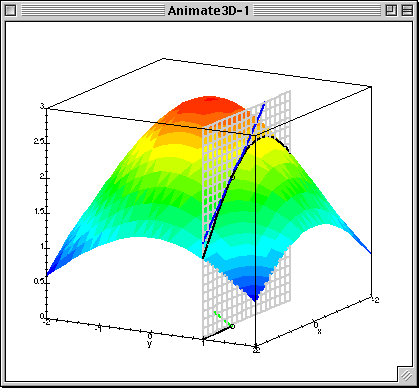Given $L(\beta_0, \beta_1)$ calculate gradient (patial derivatives) $$\frac{\partial L}{\partial \beta_0} = \frac{1}{N}\sum^{N}_{i=1}(\beta_0 + \beta_1x_{n}^1 - y^{n})$$ $$\frac{\partial L}{\partial \beta_1} = \frac{1}{N}\sum^{N}_{i=1}(\beta_0 + \beta_1x_{n}^1 - y^{n})x^1_{n}$$

Or in matrix form: $$\nabla_{\beta}L(\beta) = \frac{1}{N} X^\top(X\beta - y)$$

Run gradient update, which is simultaneous(!!!) update of $\beta$ in antigradient direction:

$$\beta := \beta - \alpha\nabla_{\beta}L(\beta)$$
• $\alpha$ - descent "speed"

## Pseudocode¶

{python}
1.function gd(X, alpha, epsilon):

2.  initialise beta

3.  do:

4.      Beta = new_beta

5.      new_Beta = Beta - alpha*grad(X, beta)

6.  until dist(new_beta, beta) < epsilon

7.  return beta
In :
grad_demo(iters=105, alpha=0.08)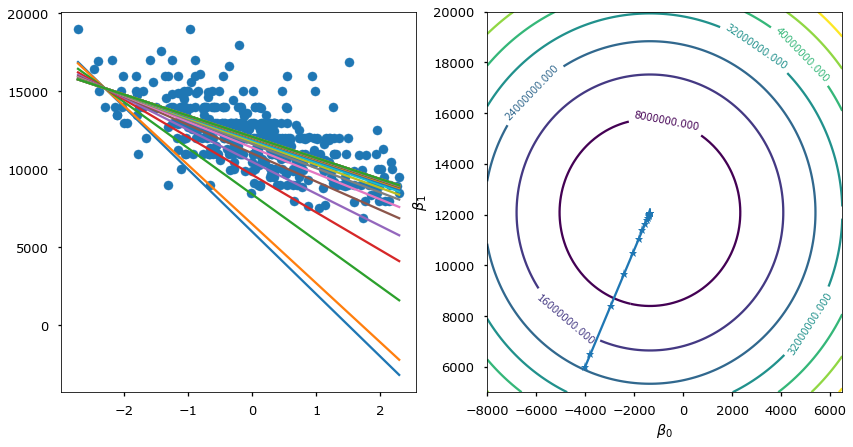## Comments¶

• How do we set $\alpha$
• Feature scales matters• Local minima*

## Gradient descent modifications¶

• Stochastic gradient descent (!)
• Descent with momentum
• Adagrad## Stochastic gradient descent¶

{python}
1.function sgd(X, alpha, epsilon):

2.  initialise beta

3.  do:

4.        X = shuffle(X)

5.        for x in X:

6.            Beta = new_beta

7.            new_Beta = Beta - alpha*grad(x, beta)

8.  until dist(new_beta, beta) < epsilon

9.  return beta
In :
stoch_grad_demo(iters=105, alpha=0.08)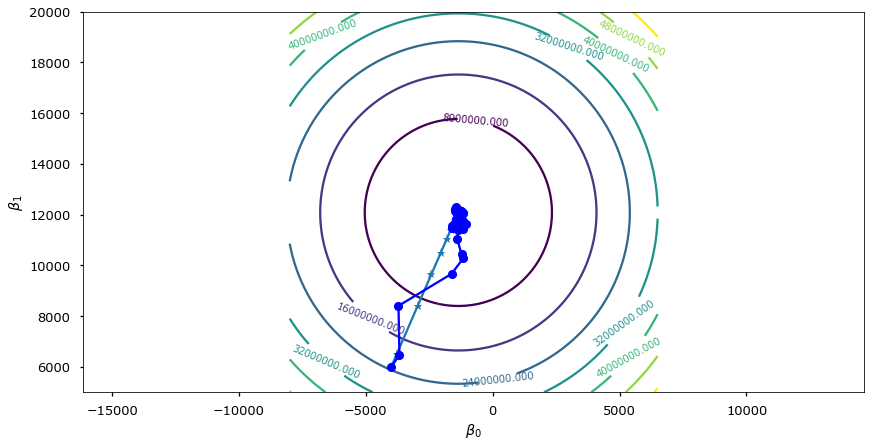### Momentum¶

Idea: to move not only to current antigradient direction but consider previous one

$$v_0 = 0$$$$v_t = \gamma v_{t - 1} + \alpha\nabla_{\beta}{L(\beta)}$$$$\beta = \beta - v_t$$

where

• $\gamma$ — momentum term (usually 0.9)### Adagrad¶

Idea: update parameters $\beta_i$ for each feature differenly.

Denote $\frac{\partial L}{\partial \beta_i}$ on iteration $t$ as $g_{t,i}$

Vanilla gd update

$$\beta_{t+1, i} = \beta_{t, i} - \alpha \cdot g_{t,i}$$

In Adagrad $\alpha$ is normalized wrt "size" of previous derivatives:

$$\beta_{t+1, i} = \beta_{t, i} - \dfrac{\alpha}{\sqrt{G_{t,ii} + \varepsilon}} \cdot g_{t,i}$$

where $G_t$ is diagonal matrix with sum of squares of derivatives of $\beta_{i}$ before iteration $t$. $\varepsilon$ — is smoothing hyperparameter.

## FYI¶

• Zero-order methods
• like...
• 2nd order methods
• Newton method

# Nonlinear dependencies¶

## Generalization by nonlinear transformations¶

Nonlinearity by $x$ in linear regression may be achieved by applying non-linear transformations to the features:

$$x\to[\phi_{0}(x),\,\phi_{1}(x),\,\phi_{2}(x),\,...\,\phi_{M}(x)]$$$$f(x)=\mathbf{\phi}(x)^{T}\beta=\sum_{m=0}^{M}\beta_{m}\phi_{m}(x)$$

The model remains to be linear in $\beta$, so all advantages of linear regression remain.

## Typical transformations¶

• $x^{i}\in[a,b]$ : binarization of feature
• $x^{i}x^{j}$ : interaction of features
• $\exp\left\{ -\gamma\left\lVert x-\tilde{x}\right\rVert ^{2}\right\}$ : closeness to reference point $\tilde{x}$
• $\ln x_{k}$ : the alignment of the distribution with heavy tails
In :
demo_weights()